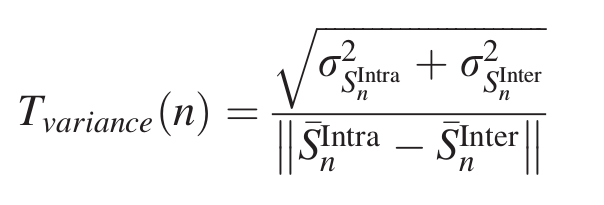# Department of Mathematics and Computer Science

www.univ-soukahras.dz/en/dept/mi

Module: Initiation à la recherche

1. Information
2. Questions

## Writing Equations using Latex (10)

 1 votes What's the latex code for the following Equation:Asked on 19:34, Monday 16 May 2016 By Imed BOUCHRIKA In Initiation à la recherche

 1 votes \begin{equation} T_{variance}(n) = \frac{\sqrt{\sigma_{S_{n}^{Intra}}^2 + {\sigma_{S_{n}^{Inter}}^2}}} {\left \| \bar S_n^{Intra} - \bar S_n^{Inter} \right \|} \end{equation} Answered on 19:54, Tuesday 17 May 2016 by Hanen Rouainia(322 points) In Initiation à la recherche

 -4 votes {begin{equation/ T_{variance}=(n) = /frac{/sqrt/sigma_{s_{n}^[intra}}^2+{/sigma_{S_{n}^{inter}}^2}}} {l/{left/l/l /bar s_n^{intra}- / bar s_n^{inter}/right  {end{equation/ Answered on 15:44, Wednesday 18 May 2016 by rahil serghine (17 points) In Initiation à la recherche

 0 votes \begin{equation}T_{variance} (n) = \frac {\sqrt {\sigma_{S_n^{Intra}}^2 + \sigma_{S_n^{Intra}}^2}} {\| \bar{S}_n^{Intra} - \bar{S}_n^{Intra} \| }\end{equation} Answered on 20:30, Friday 27 May 2016 by amirat abdallah(256 points) In Initiation à la recherche
 0 votes $T_{varience}(n)=\frac{\sqrt{\sigma _{S_{n}^{Intra}}^{2}+\sigma_{S_{n}^{Inter}}^{2}}}{\left\Vert \overline{S}_{n}^{Intra}-\overline{S}%_{n}^{Inter}\right\Vert }$ Or \begin{equation*}T_{varience}(n)=\frac{\sqrt{\sigma _{S_{n}^{Intra}}^{2}+\sigma_{S_{n}^{Inter}}^{2}}}{\left\Vert \overline{S}_{n}^{Intra}-\overline{S}%_{n}^{Inter}\right\Vert }\end{equation*} Answered on 14:24, Saturday 28 May 2016 by Mohammed BELLOUFI In Initiation à la recherche